# Rotation Around Center

Hi,

I want to rotate a Tile of my Tilemap around its center point, instead of its top left corner.

I tried some calculations with Sinus and Cosinus, but no luck… I also found that some people were talking about Matrix, which I don’t know what it is, but maybe that’s the solution?

If anyone can help me or at least point me in the right direction, I would be greatful.

Thank you!

1 Like

There are a lot of examples in the internet. Here is solution using matrix: http://stackoverflow.com/questions/15789168/as3-rotate-an-object-around-its-center-point . This is AS3, but the point should be clear. There are also other ways, including trigonometric.

It almost works. The tile starts rotating around its center, it starts floating a bit up, then stops, then move up again… It keeps like this infinitly. Here’s my code:

``````var m:Matrix = matrix.clone();
var point:Point = new Point(x + width / 2, y + height / 2);

m.translate(-point.x, -point.y);
m.rotate(rotation_value * Math.PI / 180);
m.translate(point.x, point.y);

set_matrix(m);
``````

I think the problem may be with the `width / 2` and `height / 2`.

I have smilar problemI created `TileEx` class and use it instead of `Tile`. I added width and height to id, and tried overwriting `set_rotation`

``````override private function set_rotation (value:Float):Float {
if (value != __rotation) {
__rotation = value;

var radians = value * (Math.PI / 180);

if(anchorToCenter) {
var point:Point = new Point(x + width / 2, y + height / 2);
matrix.translate(-point.x, -point.y);
matrix.translate(point.x, point.y);
} else {

var __scaleX = this.scaleX;
var __scaleY = this.scaleY;
matrix.a = __rotationCosine * __scaleX;
matrix.b = __rotationSine * __scaleX;
matrix.c = -__rotationSine * __scaleY;
matrix.d = __rotationCosine * __scaleY;
}

__transformDirty = true;
}

return value;
}
``````

But that doesn’t work at all.

My situation’s also with a Class that I created which extends Tile.

I was thinking that maybe we could try to solve this by simply analysing the rotation value. For example, At 45°, the sprite is at his lowest on Y, so we need to move the sprite up a bit. At 225°, the sprite is at his highest on Y, so we need to move it down a bit. The same thing is found on X at 135° and 315°.

Maybe I’ll try to work with that instead of continue fighting with Sinus, Cosinus and Matrix!Or maybe someday they’ll add a way to choose the anchor point to the OpenFL Tile class.

Let’s not loose hope!

I think this is worth trying. Let me provide a couple diagrams to make all of this easier to visualize.

To make this as easy as possible, let’s pick some convenient numbers. -100 as the minimum, and 100 as the maximum. So at 45°, where Y is lowest, it’s -100: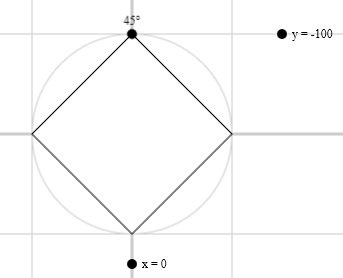At 225°, where it’s highest, Y is 100: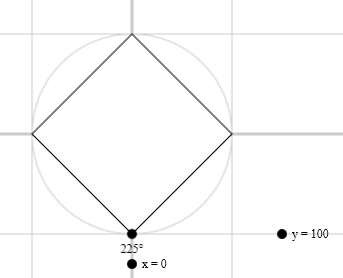(This is nothing new; I’m just illustrating your post.)

Same with X at the two other angles you mentioned: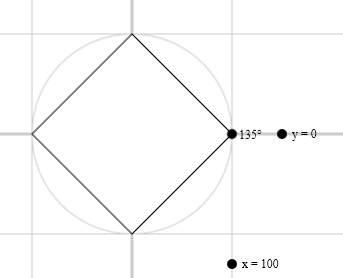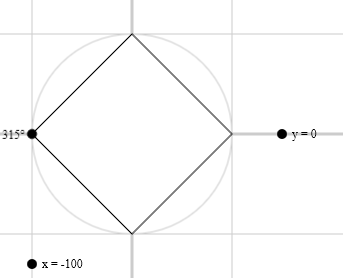So…

What X and Y values does the square have at 0°?

0° is halfway between 45° and 315°. Are the coordinates halfway between as well?

Thank you for the visuals! That’s better than the squares I drew on a piece of paper.I think you’re right about the coordinates being halfway at 0° and the same thing would happens at 90°, 180° and 270°.

I found a solution! It was simpler than I thought…

First, when the tile is created, you need to find the hypotenuse using the Pythagorean theorem:

``````var hypotenuse:Float = Math.sqrt(width / 2 * width / 2 + height / 2 * height / 2);
``````

Then, using Sinus and Cosinus, we’re able to find the center point that we want and compare it with the values of the last frame:

``````newX = hypotenuse * Math.cos((rotation + 45) * Math.PI / 180);
newY = hypotenuse * Math.sin((rotation + 45) * Math.PI / 180);

x += oldX - newX;
y += oldY - newY;

oldX = newX;
oldY = newY;
``````

I added 45 to the rotation in the equation, because at 0°, the sprite is inbetween 2 axes.

Just be sure that you have the good width and height of your sprite! That was one of my many problems…EDIT: For visual explanations, see the posts below!

Glad you figured it out! However, I had a whole thing planned, and I’d like to follow through.

Let’s take a closer look. As a reminder, here are the points at 45° and 315°.x goes from -100 to 0. If 0° is halfway between that, then x is -50. y goes from 0 to -100, so the same logic applies.

Now, let’s draw it: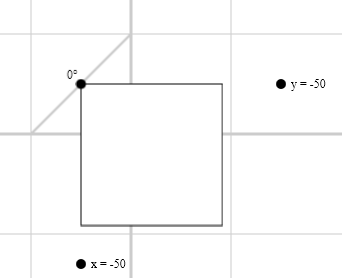That square is off-center!

Did we do the math wrong? Well, no. That diagonal line is a perfectly straight line between the points (-100, 0) and (0, -100). Our square is halfway along that line.

The halfway point isn’t what we want. It just isn’t. We want our square to trace out a circle as it rotates, not a straight line. (I actually drew this circle for you in the examples above. It’s faint, but it’s there.)

Here’s what the square should look like at 0°: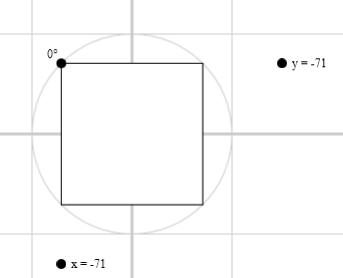See how it’s aligned with the circle? Also notice that neither x nor y equals -50.

To get a better sense of how it follows the circle, check out this interactive version.

1 Like

There’s a new (initial) implementation of `tile.originX`/`tile.originY` for OpenFL `Tilemap`

Would be happy to hear feedback on the property name, and how it works for you guysThank you a lot `player_03`! That’s a great explanation with great visuals!

I can see that saying that the coordinates at 0° is halfway is wrong. Well… it’s halfway on the circle though!And `singmajesty`, thanks for the informations! Always nice to see that OpenFL is always leveling up!I’ll test it when I’ll have the time for sure!

Yep. The angle is halfway between “up” and “left,” but the coordinates are slightly off from halfway.

So the final question is you have to ask is, if it isn’t following the obvious formula, what formula is it following?

We need a formula to take an angle and turn it into an x or y coordinate. This formula should go from 0 → 100 → 0 → -100 → 0 as you go around the circle. In addition to that, the formula should spend plenty of time near 100 and plenty of time near -100, but not much time near 0.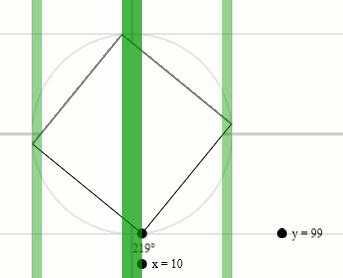This is another reason the “halfway equals halfway” strategy fails. It would spend exactly the same amount of time in each green area, and we need a formula that doesn’t.

I can clearly see the equation that I found on that gif! I’m calculating the `hypotenuse` of the triangle, which is the `radius` of the circle, to then find X and Y with Sinus and Cosinus.

Those drawings and gifs are a little bit better than my pen and paper!Thanks again!

You’re welcome!

By the way, I’m planning to turn these posts into a blog post at some point. Do you think there’s anything else I should include, or any parts I should skip?

The only thing I’m thinking is maybe add a concrete example with a sprite. In this example, you could put a triangle like in this example you shared and rotate the sprite calculating its X and Y.

And I don’t think you should skip anything. There are a lot of good informations!@player_03 have you complete example how you implemented it.
I have project where I need center rotation

Mallario posted the math here.

@player_03 yes I sow this, but no luck ((( my goal is to center rotate TextField, could’t figure out how to do that (((

The math should work the same. If it won’t display angled text at all, that’s probably an issue with the platform, not with the formula.

What have you tried, and what happens when you try?

simply replaced @Mallario formula

``````var oldX = flatReservedTxt.x;
var oldY = flatReservedTxt.y;

var hypotenuse:Float = Math.sqrt(flatReservedTxt.width / 2 * flatReservedTxt.width / 2 + flatReservedTxt.height / 2 * flatReservedTxt.height / 2);
var newX = hypotenuse * Math.cos((flatReservedTxt.rotation + 45) * Math.PI / 180);
var newY = hypotenuse * Math.sin((flatReservedTxt.rotation + 45) * Math.PI / 180);

flatReservedTxt.x += oldX - newX;
flatReservedTxt.y += oldY - newY;

oldX = newX;
oldY = newY;
``````

am I missing something? and what is number 45 degree?